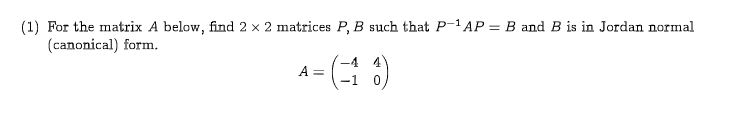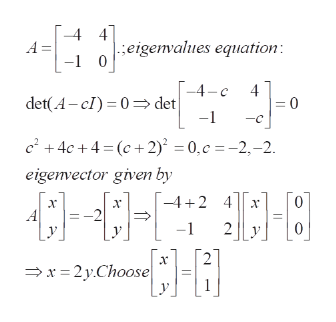# (1) For the matrix A below, find 2 x 2 matrices P, B such that P-1 AP(canonical) form.B and B is in Jordan normal-4 4(0)A =

Question

Linear algebrahelp_outlineImage Transcriptionclose(1) For the matrix A below, find 2 x 2 matrices P, B such that P-1 AP (canonical) form. B and B is in Jordan normal -4 4 (0) A = fullscreen
check_circle

Step 1

To transform the given matrix into Jordan canonical form

Step 2

Eigenvalues and eigenvectors of the given matrix A; note that the eigenvalue -2 is repeated (twice) but there is only one linearly independent eigenvector. Therefore the matrix A is not diagonalizable.help_outlineImage Transcriptionclose4] eigenvalues equation -1 0 4 A -4-c 4 det(A-cI) 0>det -1 c+4c+4 (c+2) = 0,c =-2,-2 eigenvector given by -42 4 х х -1 х x2yChoose fullscreen
Step 3

To find the matrix P, solve the gene...

### Want to see the full answer?

See Solution

#### Want to see this answer and more?

Solutions are written by subject experts who are available 24/7. Questions are typically answered within 1 hour.*

See Solution
*Response times may vary by subject and question.
Tagged in

### Math